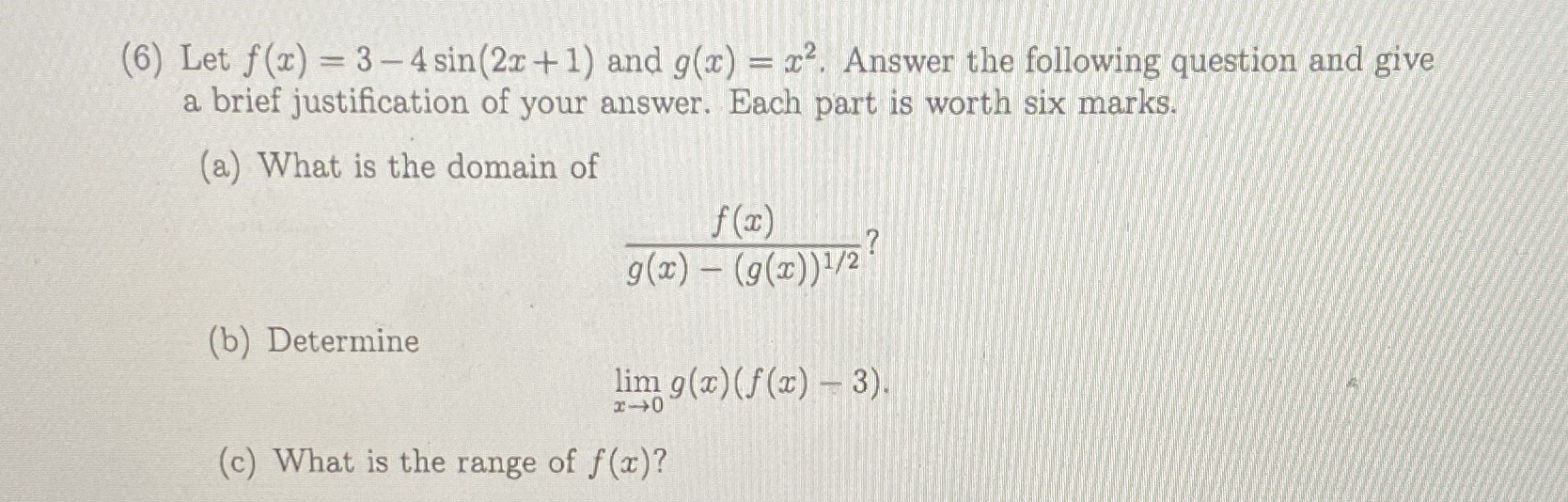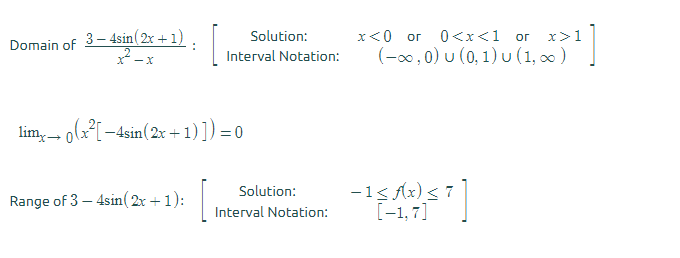### ¿Todavía tienes preguntas de matemáticas?

Pregunte a nuestros tutores expertos
Algebra
Pregunta(6) Let $$f ( x ) = 3 - 4 \sin ( 2 x + 1 )$$ and $$g ( x ) = x ^ { 2 } .$$ Answer the following question and give a brief justification of your answer. Each part is worth six marks.

(a) What is the domain of

(b) Determine $$\frac { f ( x ) } { g ( x ) - ( g ( x ) ) ^ { 1 / 2 } } ?$$

(c) What is the range of $$f ( x ) ?$$